# 100 In Binary

In math, we follow a standard form to represent a number. We call it the number system.

We have different types of number systems in Math.

The binary number system is one of the most common systems to be used. This system uses only two digits $$0$$ and $$1$$

Thus, the numbers in this system have a base 2

0 and 1 are called bits.

Let us find an answer to the question what is 100 in binary?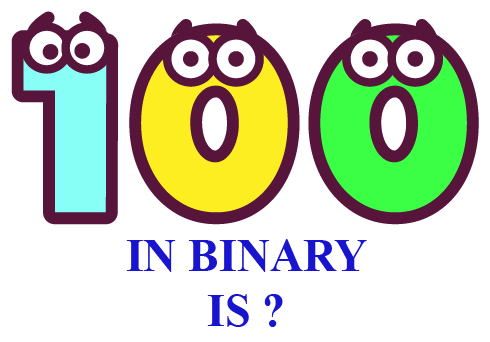In this mini lesson, we will explore the world of $$100_{10}$$ in binary. We will walk through the answers to the questions like what is meant by converting $$100_{10}$$ from decimal to binary, and how to convert $$100_{10}$$ from decimal to binary number.

## Lesson Plan

 1 What Is Meant By Converting 100 From Decimal to Binary? 2 Important Notes on 100 I Binary 3 Solved Examples 4 Interactive Questions on 100 In Binary 5 Challenging Question on 100 In Binary

## What Is Meant By Converting 100 From Decimal to Binary?

$$100_{10}$$ is a decimal representation. Converting 100 from decimal to binary means to write or represent 100 using 2 bits only, i.e., 0 and 1.

In converting 100 in binary, we need to change the base 10 to base 2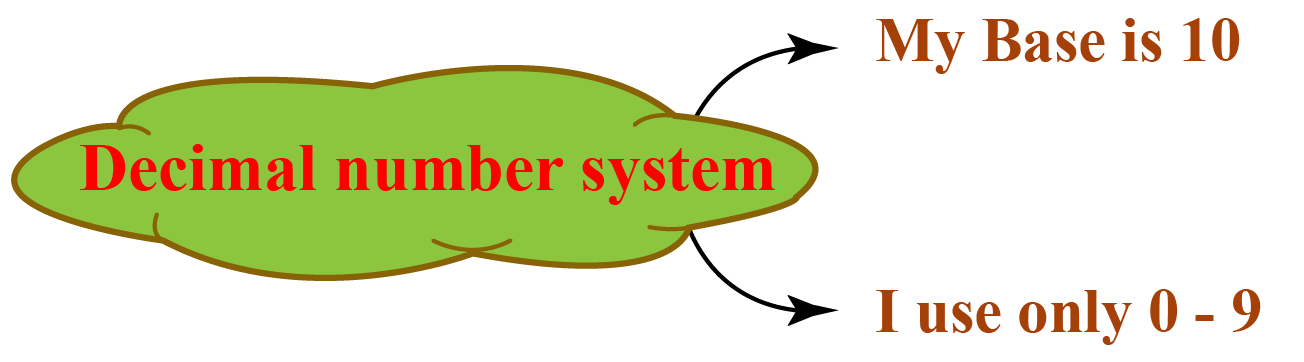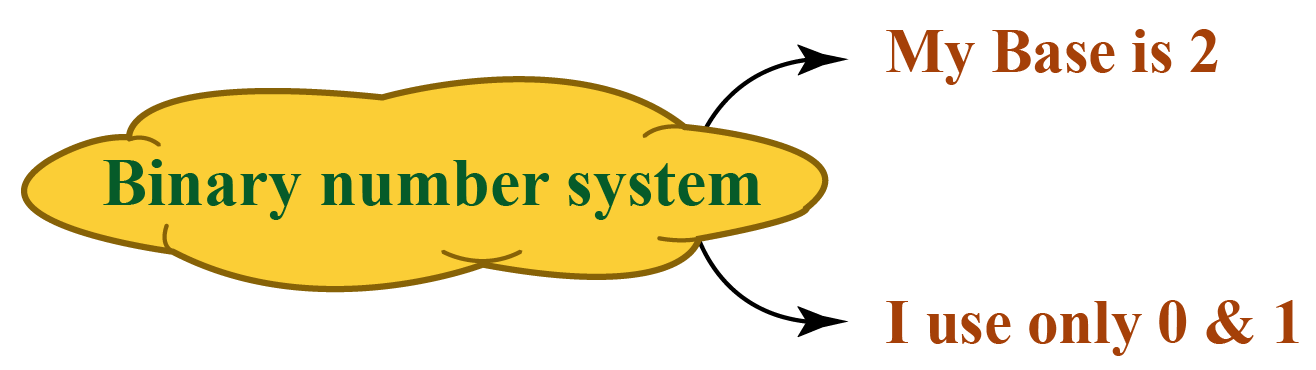$$100_{10}$$ in binary is $$1100100_{2}$$

## How to Convert 100 From Decimal to Binary Number?

Let us look at the steps showing the conversion of 100  from decimal to binary.

Let us look at the steps showing the conversion of 100 from decimal to binary.

Step-1

Identify the base of the required number. In this case, the base of 100 is 10, i.e., $$100_{10}$$

Step-2

Divide the given number 100 by the base of the required number, i.e. 2

Step-3

Note down the quotient and the remainder.

Step-4

Divide the number 100 until we get the quotient to be less than the base which is 2

Step-5

Read all the remainders including the last quotient from bottom to top.

(Note: digits marked in the green boxes represent the binary form of 100.)

Repeat this process (dividing the quotient again by the base) until we get the quotient to be less than the base which is 2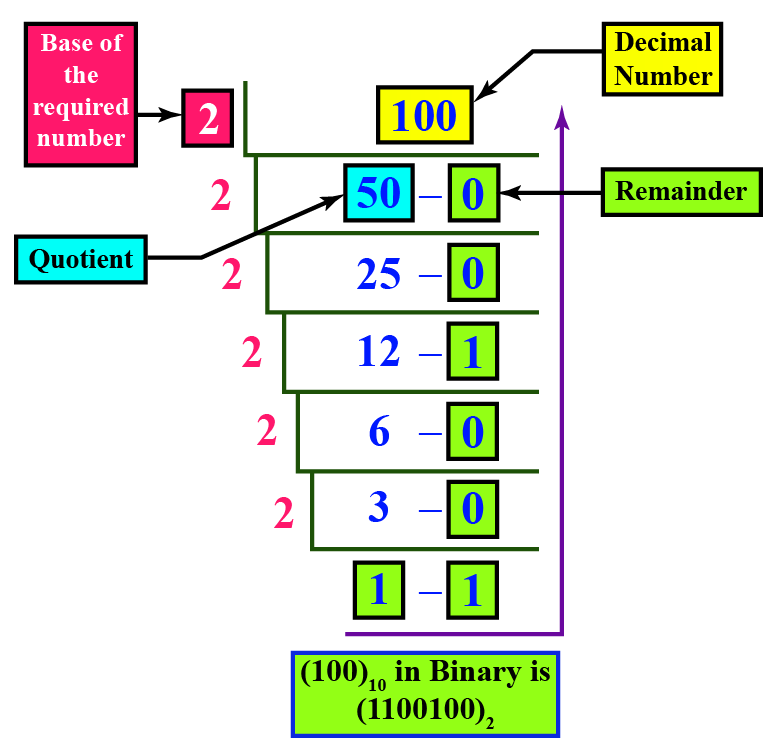Therefore  $$100_{10} = 1100100_2$$

### Binary to Decimal Conversion

Step 1: Identify the base of the given number.

Here, the base of $$100111_2$$ is 2

Step 2: Multiply each digit of the given number, starting from the rightmost digit, with the exponents of the base.

The exponents should start with 0 and increase by 1 every time as we move from right to left.

Since the base here is 2, we multiply the digits of the given number by $$2^0, 2^1, 2^2,...$$ from right to left.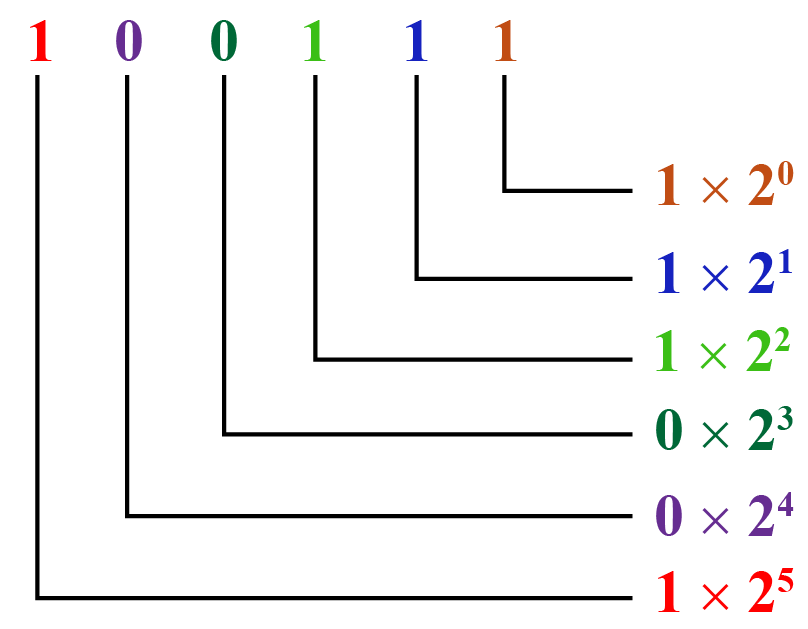Step 3: We just simplify each of the above products and add them.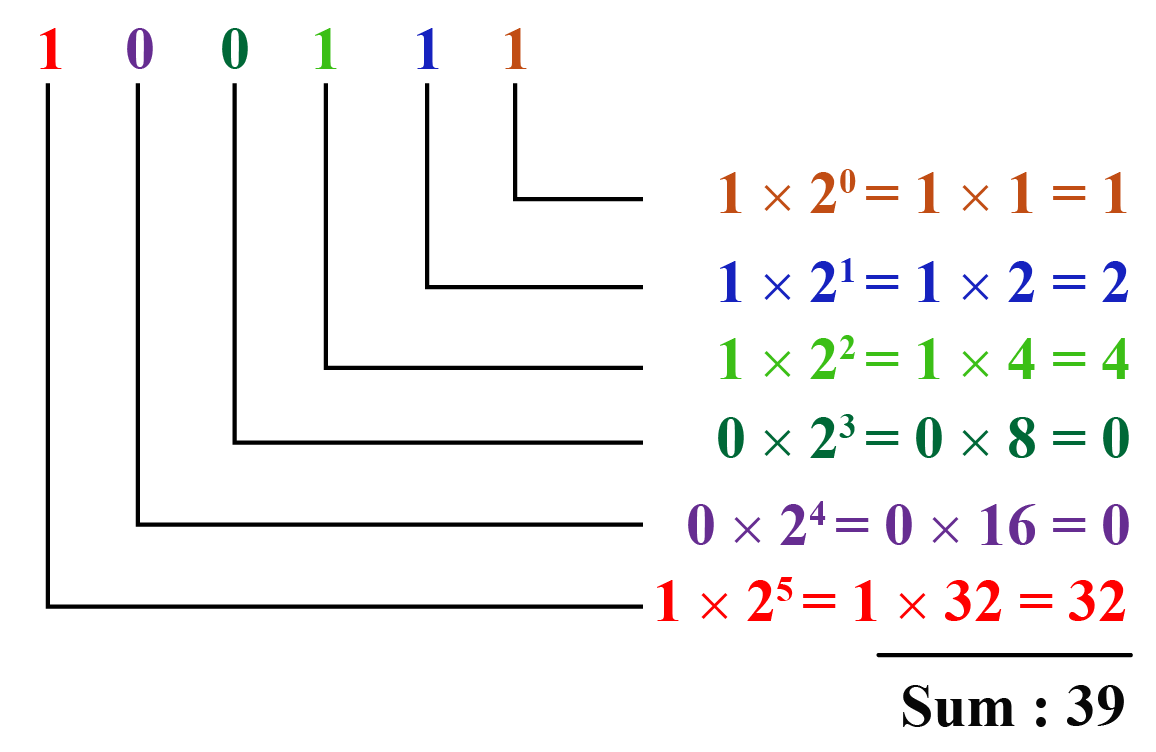Here, the sum is the equivalent number in the decimal number system of the given number.

$$\therefore 100111_2 = 39_{10}$$

### Decimal to Binary Converter.

Try to convert some more decimal numbers into binary using decimal to binary converter.

More Important Topics
Numbers
Algebra
Geometry
Measurement
Money
Data
Trigonometry
Calculus